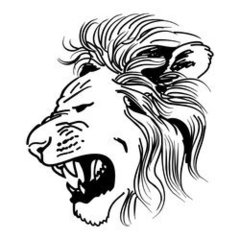# ssget filter## Recommended PostsHow can I add a filter in ssget...? Actually, I want to select only unlocked line with cross selection. is it possible with this": L"

`(setq lines (ssget "_C" p1 p2 (list (cons 0 "LINE"))))`

like this

`(ssget "_+.:S:E:L" '((0 . "ARC,CIRCLE,ELLIPSE,LINE")))`

this is not getting how. can i add can someone help

`(ssget "_+.c:L" '((0 . "ARC,CIRCLE,ELLIPSE,LINE")))`

i want to edit this lisp

```(setq p1 (getpoint "\nSelect object..."))
(setq p2 (getcorner p1))
(setq mp (polar p1 (angle p1 p2) (/ (distance p1 p2) 2)))
(setq p1 (list (nth 0 p1) (nth 1 p1)))
(setq p2 (list (nth 0 p2) (nth 1 p2)))
(setq lines (ssget "_C" p1 p2 (list (cons 0 "LINE"))))
(if (/= (sslength lines) 4)
(alert "4 lines need to be selected")```

Edited by Ajmal
##### Share on other sitesLee Mac has a great website for explaining the ssget filters. Here is the link.

##### Share on other sitesTry this

`(if (not (equal '(nil nil) (sssetfirst nil (ssget "_C" p1 p2 '((0 . "LINE")))))) (setq lines (ssget "_:L")))`

##### Share on other sites22 minutes ago, dlanorh said:

Try this

```
(if (not (equal '(nil nil) (sssetfirst nil (ssget "_C" p1 p2 '((0 . "LINE")))))) (setq lines (ssget "_:L")))```

this is working. thanks.## Join the conversation

You can post now and register later. If you have an account, sign in now to post with your account.
Note: Your post will require moderator approval before it will be visible.×   Pasted as rich text.   Restore formatting

Only 75 emoji are allowed.